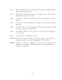## Efficient Minimum Cost Matching and Transportation Using Quadrangle Inequality

 dc.contributor.author Aggarwal, Alok en_US dc.contributor.author Bar-Noy, Amotz en_US dc.contributor.author Khuller, Samir en_US dc.contributor.author Kravets, Dina en_US dc.contributor.author Schieber, Baruch en_US dc.date.accessioned 2004-05-31T22:24:45Z dc.date.available 2004-05-31T22:24:45Z dc.date.created 1993-12 en_US dc.date.issued 1998-10-15 en_US dc.description.abstract We present efficient algorithms for finding a minimum cost perfect matching, and for solving the transportation problem in bipartite graphs, G=(\Red\cup \Blue, \Red\times \Blue), where |\Red|=n, |\Blue|=m, n\le m, and the cost function obeys the quadrangle inequality. First, we assume that all the \red\ points and all the \blue\ points lie on a curve that is homeomorphic to either a line or a circle and the cost function is given by the Euclidean distance along the curve. We present a linear time algorithm for the matching problem that is simpler than the algorithm of \cite{kl75}. We generalize our method to solve the corresponding transportation problem in O((m+n) \log (m+n)) time, improving on the best previously known algorithm of \cite{kl75}. Next, we present an O(n\log m)-time algorithm for minimum cost matching when the cost array is a bitonic Monge array. An example of this is when the \red\ points lie on one straight line and the \blue\ points lie on another straight line Finally, we provide a weakly polynomial algorithm for the transportation problem in which the associated cost array is a bitonic Monge array. Our algorithm for this problem runs in O(m \log(\sum_{j=1}^m \sj_j)) time, where \di_i is the demand at the ith sink, \sj_j is the supply available at the jth source, and \sum_{i=1}^n \di_i \le \sum_{j=1}^m \sj_j. (Also cross-referenced as UMIACS-TR-93-140) en_US dc.format.extent 414595 bytes dc.format.mimetype application/postscript dc.identifier.uri http://hdl.handle.net/1903/610 dc.language.iso en_US dc.relation.isAvailableAt Digital Repository at the University of Maryland en_US dc.relation.isAvailableAt University of Maryland (College Park, Md.) en_US dc.relation.isAvailableAt Tech Reports in Computer Science and Engineering en_US dc.relation.isAvailableAt UMIACS Technical Reports en_US dc.relation.ispartofseries UM Computer Science Department; CS-TR-3199 en_US dc.relation.ispartofseries UMIACS; UMIACS-TR-93-140 en_US dc.title Efficient Minimum Cost Matching and Transportation Using Quadrangle Inequality en_US dc.type Technical Report en_US
##### Original bundle
Now showing 1 - 2 of 2Name:
CS-TR-3199.ps
Size:
404.88 KB
Format:
Postscript Files
Description: